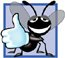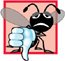# Increment and Decrement Operators

In addition to the arithmetic assignment operators, C++ also provides two unary operators for adding 1 to or subtracting 1 from the value of a numeric variable. These are the unary increment operator, ++, and the unary decrement operator, --, which are summarized in Fig. 4.20. A program can increment by 1 the value of a variable called c using the increment operator, ++, rather than the expression c = c + 1 or c += 1. An increment or decrement operator that is prefixed to (placed before) a variable is referred to as the prefix increment or prefix decrement operator, respectively. An increment or decrement operator that is postfixed to (placed after) a variable is referred to as the postfix increment or postfix decrement operator, respectively.

Figure 4.20. Increment and decrement operators.

Operator

Called

Sample expression

Explanation

++

preincrement

++a

Increment a by 1, then use the new value of a in the expression in which a resides.

++

postincrement

a++

Use the current value of a in the expression in which a resides, then increment a by 1.

--

predecrement

--b

Decrement b by 1, then use the new value of b in the expression in which b resides.

--

postdecrement

b--

Use the current value of b in the expression in which b resides, then decrement b by 1.

Using the prefix increment (or decrement) operator to add (or subtract) 1 from a variable is known as preincrementing (or predecrementing) the variable. Preincrementing (or predecrementing) causes the variable to be incremented (decremented) by 1, and then the new value of the variable is used in the expression in which it appears. Using the postfix increment (or decrement) operator to add (or subtract) 1 from a variable is known as postincrementing (or postdecrementing) the variable. Postincrementing (or postdecrementing) causes the current value of the variable to be used in the expression in which it appears, and then the variable's value is incremented (decremented) by 1.

Good Programming Practice 4.8Unlike binary operators, the unary increment and decrement operators should be placed next to their operands, with no intervening spaces.

Figure 4.21 demonstrates the difference between the prefix increment and postfix increment versions of the ++ increment operator. The decrement operator (--) works similarly. Note that this example does not contain a class, but just a source code file with function main performing all the application's work. In this chapter and in Chapter 3, you have seen examples consisting of one class (including the header and source code files for this class), as well as another source code file testing the class. This source code file contained function main, which created an object of the class and called its member functions. In this example, we simply want to show the mechanics of the ++ operator, so we use only one source code file with function main. Occasionally, when it does not make sense to try to create a reusable class to demonstrate a simple concept, we will use a mechanical example contained entirely within the main function of a single source code file.

Figure 4.21. Preincrementing and postincrementing.

(This item is displayed on page 163 in the print version)

``` 1 // Fig. 4.21: fig04_21.cpp
2 // Preincrementing and postincrementing.
3 #include
4 using std::cout;
5 using std::endl;
6
7 int main()
8 {
9 int c;
10
11 // demonstrate postincrement
12 c = 5; // assign 5 to c
13 cout << c << endl; // print 5
14 cout << c++ << endl; // print 5 then postincrement
15 cout << c << endl; // print 6
16
17 cout << endl; // skip a line
18
19 // demonstrate preincrement
20 c = 5; // assign 5 to c
21 cout << c << endl; // print 5
22 cout << ++c << endl; // preincrement then print 6
23 cout << c << endl; // print 6
24 return 0; // indicate successful termination
25 } // end main
```

 ``` 5 5 6 5 6 6 ```

Line 12 initializes the variable c to 5, and line 13 outputs c's initial value. Line 14 outputs the value of the expression c++. This expression postincrements the variable c, so c's original value (5) is output, then c's value is incremented. Thus, line 14 outputs c's initial value (5) again. Line 15 outputs c's new value (6) to prove that the variable's value was indeed incremented in line 14.

Line 20 resets c's value to 5, and line 21 outputs c's value. Line 22 outputs the value of the expression ++c. This expression preincrements c, so its value is incremented, then the new value (6) is output. Line 23 outputs c's value again to show that the value of c is still 6 after line 22 executes.

The arithmetic assignment operators and the increment and decrement operators can be used to simplify program statements. The three assignment statements in Fig. 4.17

```passes = passes + 1;
failures = failures + 1;
studentCounter = studentCounter + 1;
```

can be written more concisely with assignment operators as

```passes += 1;
failures += 1;
studentCounter += 1;
```

with prefix increment operators as

```++passes;
++failures;
++studentCounter;
```

or with postfix increment operators as

```passes++;
failures++;
studentCounter++;
```

Note that, when incrementing (++) or decrementing (--) of a variable occurs in a statement by itself, the preincrement and postincrement forms have the same effect, and the predecrement and postdecrement forms have the same effect. It is only when a variable appears in the context of a larger expression that preincrementing the variable and postincrementing the variable have different effects (and similarly for predecrementing and postdecrementing).

Common Programming Error 4.14Attempting to use the increment or decrement operator on an expression other than a modifiable variable name or reference, e.g., writing ++(x + 1), is a syntax error.

Figure 4.22 shows the precedence and associativity of the operators introduced to this point. The operators are shown top-to-bottom in decreasing order of precedence. The second column indicates the associativity of the operators at each level of precedence. Notice that the conditional operator (?:), the unary operators preincrement (++), predecrement (--), plus (+) and minus (-), and the assignment operators =, +=, -=, *=, /= and %= associate from right to left. All other operators in the operator precedence chart of Fig. 4.22 associate from left to right. The third column names the various groups of operators.

Figure 4.22. Operator precedence for the operators encountered so far in the text.

Operators

Associativity

Type

()

left to right

parentheses

++

--

static_cast< type >()

left to right

unary (postfix)

++

--

+

-

right to left

unary (prefix)

*

/

%

left to right

multiplicative

+

-

left to right

<<

>>

left to right

insertion/extraction

<

<=

>

>=

left to right

relational

==

!=

left to right

equality

?:

right to left

conditional

=

+=

-=

*=

/=

%=

right to left

assignmentC++ How to Program (5th Edition)
ISBN: 0131857576
EAN: 2147483647
Year: 2004
Pages: 627

Similar book on Amazon